﻿ 基于从头计算势能面的H + HS反应的理论研究 Theoretical Study of the Reaction H + HS Based on the Ab initio Potential Energy Surface

Applied Physics
Vol. 09  No. 01 ( 2019 ), Article ID: 28447 , 7 pages
10.12677/APP.2019.91004

Theoretical Study of the Reaction H + HS Based on the Ab initio Potential Energy Surface

Yuliang Wang*, Dianyu Gu, Hui Li, Kun Wang

School of Basic Sciences for Aviation, Naval Aviation University, Yantai Shandong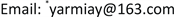Received: Dec. 23rd, 2018; accepted: Jan. 7th, 2019; published: Jan. 14th, 2019ABSTRACT

Quasi-classical trajectory (QCT) method has been used to calculate the dynamics of the reaction H + HS→S + H2 on the newly developed potential energy surface of lowest triplet state. The agreement of reaction probability and integral cross section between QCT and time-dependent wave packet (TDWP) calculations are fairly good. The results suggested that the collision energy enhances the reactivity in the low collision energy range, whereas inhibits the reaction at relatively high energies. In addition, the product molecules H2 are mainly scattered forwards and sideways, and the scattering intensity and direction are sensitive to the collision energy.

Keywords:Quasi-Classical Trajectory, Stereodynamics, Vector Correlations1. 引言

2. 理论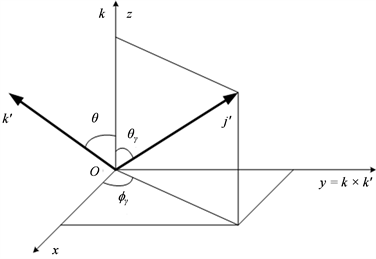Figure 1. The xyz center-of-mass (CM) reference frame describing the vector correlations

$P\left(\mathrm{cos}\theta ,\text{cos}{\theta }_{r},{\varphi }_{r}\right)=\underset{k=0}{\overset{\infty }{\sum }}\underset{q=-k}{\overset{k}{\sum }}\frac{2k+1}{4\text{π}}×\left(\frac{2\text{π}}{\sigma }\frac{\text{d}{\sigma }_{kq}}{\text{d}\omega }\right){C}_{kq}^{*}\left({\theta }_{r},{\varphi }_{r}\right)$

$P\left({\varphi }_{r}\right)=\frac{1}{\text{2π}}\left(1+\underset{even,n\ge 2}{\sum }{a}_{n}\mathrm{cos}n{\varphi }_{r}+\underset{even,n\ge 1}{\sum }{b}_{n}\mathrm{sin}n{\varphi }_{r}\right)$

$P\left({\theta }_{r}\right)=\frac{1}{2}\underset{k}{\sum }\left(2k+1\right){a}_{0}^{\left(k\right)}{P}_{k}\left(\mathrm{cos}{\theta }_{r}\right)$

${a}_{0}^{\left(k\right)}=\underset{0}{\overset{\text{π}}{\int }}P\left({\theta }_{r}\right){P}_{k}\left(\mathrm{cos}{\theta }_{r}\right)\mathrm{sin}{\theta }_{r}\text{d}{\theta }_{r}=〈{P}_{k}\left(\mathrm{cos}{\theta }_{r}\right)〉$

3. 结果和讨论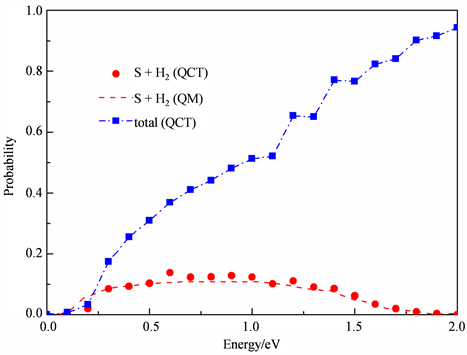Figure 2. Comparison of the reaction probabilities between the QCT and TDWP calculations over the collision energy range of 0 to 2.0 eV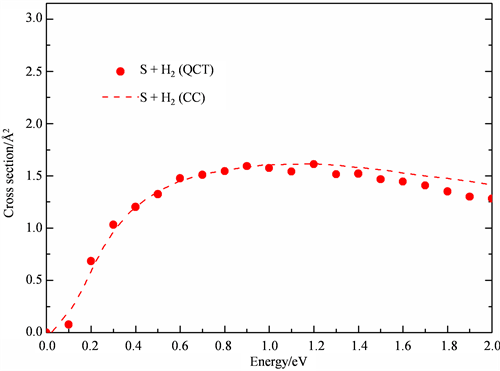Figure 3. Comparison of the integral cross sections between the QCT and CC calculations over the collision energy range of 0 to 2.0 eV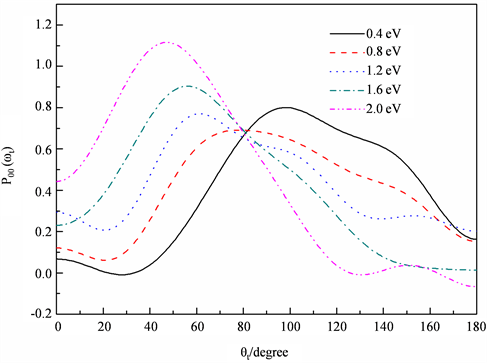Figure 4. The PDDCS P00 for the reaction at five different collision energies as indicated in the figure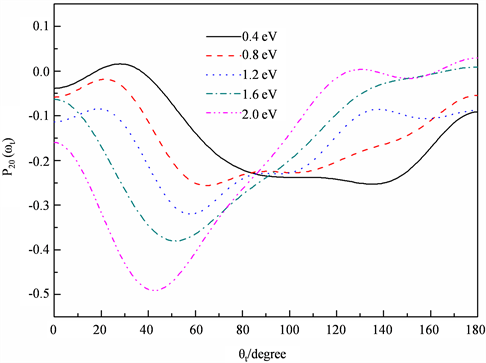Figure 5. The PDDCS P20 for the reaction at five different collision energies as indicated in the figure

4. 结论

Theoretical Study of the Reaction H + HS Based on the Ab initio Potential Energy Surface[J]. 应用物理, 2019, 09(01): 23-29. https://doi.org/10.12677/APP.2019.91004

1. 1. Martin, R.L. (1983) A Theoretical Study of the Reaction H + HS→H2 + S. Chemical Physics, 82, 337-341. https://doi.org/10.1016/0301-0104(83)85238-0

2. 2. Maiti, B., Schatz, G.C. and Lendvay, G. (2004) Importance of Intersystem Crossing in the S(3P,1D) + H2→SH + H reaction. The Journal of Physical Chemistry A, 108, 8772-8781. https://doi.org/10.1021/jp049143o

3. 3. Berteloite, C., Lara, M., Bergeat, A., et al. (2010) Kinetics and Dynamics of the Reaction S(1D) + H2→SH + H at Very Low Temperatures and Collision Energies. Physical Review Letters, 105, 203201-203204. https://doi.org/10.1103/PhysRevLett.105.203201

4. 4. Zyubin, A.S., Mebel, A.M., Chao, S.D. and Skodje, R.T. (2001) Reaction Dynamics of S(1D) + H2/D2 on a New Ab initio Potential Surface. Journal of Chemical Physics, 114, 320-330. https://doi.org/10.1063/1.1329887

5. 5. Ho, T.S., et al. (2002) A Globally Smooth Ab initio Potential Surface of the 1A' State for the Reaction S(1D) + H2. Journal of Chemical Physics, 116, 4124-4134. https://doi.org/10.1063/1.1431280

6. 6. Lv, S.J., Zhang, P.Y., Han, K.L. and He, G.Z. (2012) Exact Quantum Scattering Study of the H + HS Reaction on a New Ab initio Potential Energy Surface H2S(3A''). Journal of Chemical Physics, 136, Article ID: 094308. https://doi.org/10.1063/1.3690051

7. 7. Kandel, S.A., Alexander, A.J., et al. (2000) Cl + HD(v = 1; j = 1,2) Reac-tion Dynamics: Comparison between Theory and Experiment. Journal of Chemical Physics, 112, 670-685. https://doi.org/10.1063/1.480602

8. 8. Li, W.L., Wang, M.S., et al. (2007) Theoretical Study of the Stereodynamics of the Reactions D + H2→H + HD and H + D2→D + HD. Chemical Physics, 337, 93-98. https://doi.org/10.1016/j.chemphys.2007.06.041

NOTES

*通讯作者。